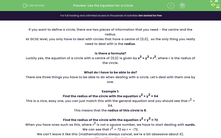# Use the Equation for a Circle

In this worksheet, students will solve problems for circles with centres of (0,0)Key stage:  KS 4

Year:  GCSE

GCSE Subjects:   Maths

GCSE Boards:   AQA, Eduqas, OCR, Pearson Edexcel,

Curriculum topic:   Algebra, Graphs of Equations and Functions

Curriculum subtopic:   Graphs Graphs of Equations and Functions

Popular topics:   Square Numbers worksheets

Difficulty level:#### Worksheet Overview

If you want to define a circle, there are two pieces of information that you need - the centre and the radius.

At GCSE level, you only have to deal with circles that have a centre of (0,0),  so the only thing you really need to deal with is the radius.

Is there a formula?

Luckily yes, the equation of a circle with a centre of (0,0) is given by x2 + y2 = r2, where r is the radius of the circle.

What do I have to be able to do?

There are three things you have to be able to do when dealing with a circle. Let’s deal with them one by one.

Example 1:

Find the radius of the circle with the equation x2 + y2 = 64

This is a nice, easy one, you can just match this with the general equation and you should see that r2 = 64.

This means that the radius of this circle is 8.

Find the radius of the circle with the equation x2 + y2 = 72

When you have ones such as this, where r2 is not a square number, we have to start dealing with surds.

We can see that r2 = 72 so r = √72.

We can’t leave it like this (mathematicians always cancel, we’re a bit obsessive about it).

√72 = √36 x  √2 = 6√2

So our radius, in its simplest form is r = 6√2

Example 2:

Is the point (3,2) inside, outside or on the circle with equation x2 + y2 = 12?

With this, we need to think about the distance between the centre of the circle (0,0) and the point (3,2).

The only way we can do this is by using Pythagoras' Theorem:

x2 = 32 + 22 → x = √13

We can now look at the radius of the circle:

r2 = 12 → r = √12

We can now see that the distance between the point and the centre of the circle (√13) is larger than the radius of the circle (√12) so the point (3,2) must be outside the circle.

Example 3:

Give a point (A) with integer coordinates that sits on the circle x2 + y2 = 20

While we’re only asked for one, there are actually eight that you could give as an answer.

The trick here is to look at the square numbers and find two that add to 20.

In this case, the numbers are 4 and 16.

We can therefore say that if x = 2 and y = 4 then x2 + y2 = 20

So point A is (2,4)

To find the other seven we need to look into a few facts:

It doesn’t matter which way round you add things, which means that x and y can just be the other way round. Point B is (4,2)

Squaring a negative number gives a positive.

This will mean that the coordinates could just be reversed:

Point C is (-2,-4) and point D is (-4,-2)

You could also have a combination of positives and negatives:

(-2,4) (2,-4) (-4,2) (4,-2)

For this question any of the ones above would be sufficient to get the mark.

Let's have a go at some questions now.

### What is EdPlace?

We're your National Curriculum aligned online education content provider helping each child succeed in English, maths and science from year 1 to GCSE. With an EdPlace account you’ll be able to track and measure progress, helping each child achieve their best. We build confidence and attainment by personalising each child’s learning at a level that suits them.

Get started